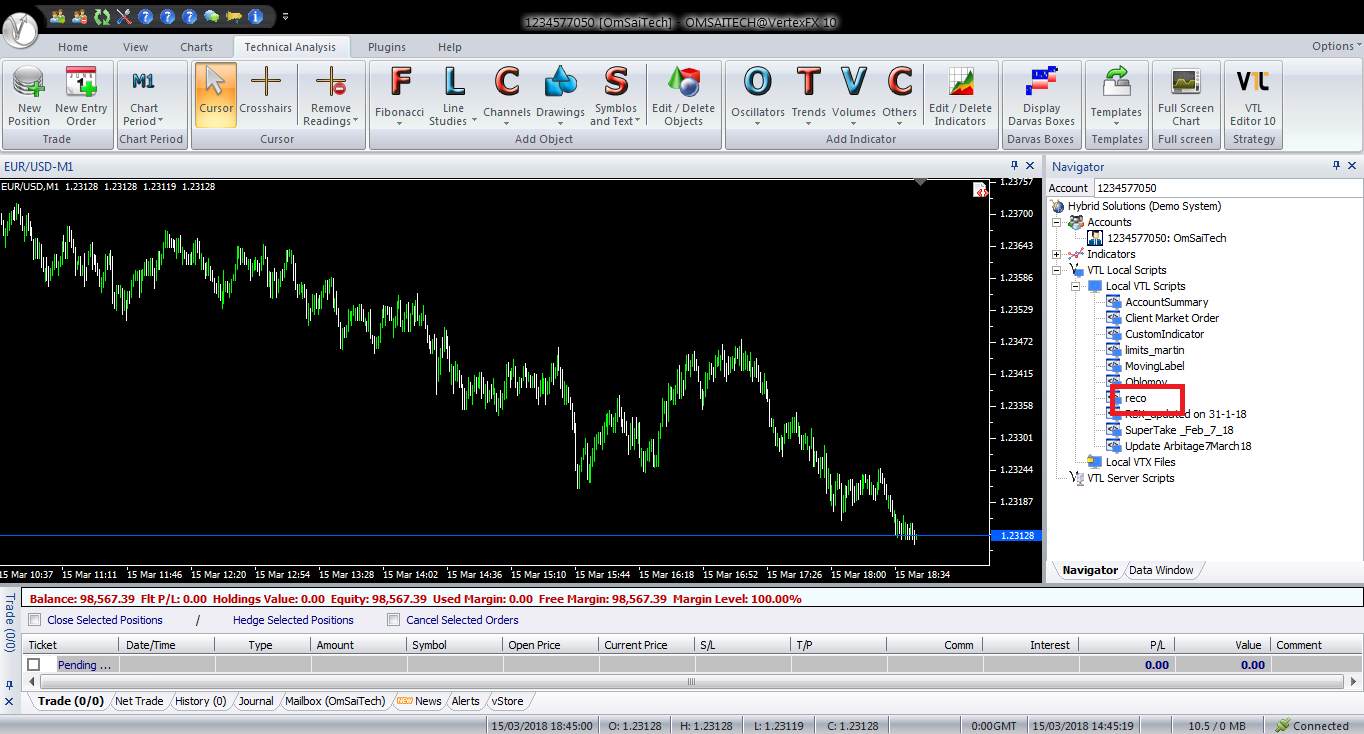# RECO

Free

Developed By: OmSaiTech

In stock

RECO Auto Trader is a VertexFX client-side script that places trades by identifying the market trend. It is based on the Relative Strength Index (RSI) oscillator and employs a variation of the Martingale based position management.

SKU: 1455 Categories: ,RECO Auto Trader is a VertexFX client-side script that places trades by identifying the market trend. It is based on the Relative Strength Index (RSI) oscillator and employs a variation of the Martingale based position management.

When the Auto Trader starts afresh without any open positions, it waits for the RSI to cross below the RSI_BUY_ZONE or above the RSI_SELL_ZONE threshold levels. The initial BUY trade is placed when the RSI is below the RSI_BUY_ZONE, and likewise, the initial SELL trade is placed when the RSI is above the RSI_SELL_ZONE.

A similar contrary rule is employed to place SELL trades, where the next SELL trade is at a farther distance above the previous SELL trade calculated from START_DISTANCE and DISTANCE_MULTIPLIER with increasing lot size.
This mechanism adds safety to the Auto Trader as it ensures that the new positions are not opened very quickly. This is especially valuable when the market is falling (or rising) very quickly, whereby the Auto Trader avoids adding new trades very quickly and risking ruin.

RECO Auto Trader does not use profit target and stop-loss for individual trades. It uses combined net profit / loss to exit the open positions when the profit or loss threshold is crossed. The profit threshold is calculated if USE_CLOSE_PROFIT is set TRUE, and likewise the loss threshold is calculated if USE_CLOSE_LOSE is set to TRUE. The calculations are as follows,

Profit Threshold = PROFIT_1_ORDER x PROFIT_MULTIPLIER positions – 1
Loss Threshold = LOSE_1_ORDER x LOSE_MULTIPLIER positions – 1
To illustrate, assume PROFIT_1_ORDER is set to 3, PROFIT_MULTIPLIER is set to 2. If only one trade is open, it will be closed when the profit reaches \$3, if two trades are open then both trades will be closed when the combined profit reaches \$6, if three trades are open then these three trades will be closed when the combined profit reaches \$12. The loss threshold can be calculated similarly from the above example by substituting the respective values of LOSE_1_ORDER and LOSE_MULTIPLIER.

Configurable Inputs

1.MAX_ORDERS – The maximum number of trades that can be opened by this Auto Trader.

2.MAGIC– The unique number that identifies trades generated by this Auto Trader.

3.RSI_PERIOD–The period used to calculate the Relative Strength Index (RSI).

4.RSI_SELL_ZONE–The RSI threshold value above which the Auto Trader will initiate SELL orders. For example, if this value is set to 70, the Auto Trader will open SELL orders when the RSI is 70 or above.

5.RSI_BUY_ZONE– The RSI threshold value below which the Auto Trader will initiate BUY orders. For example, if this value is set to 30, the Auto Trader will open BUY orders when the RSI is 30 or below.

6.LOT – The lot-size of the first (initial) trade opened by the Auto Trader.

7.LOT_MULTIPLIER –The multiplier applied to the previous lot-size for each subsequent trade.
8.MIN_LOT –The minimum allowed lot-size of any given trade. If this value is set to 0, then it is ignored.

9.MAX_LOT –The maximum allowed lot-size of any given trade. If this value is set to 0, then it is ignored.

10.START_DISTANCE –The distance in points of the second trade from the first trade. For example, if this value is set to 50, then the second BUY trade is opened at a distance of at least 50 points below the first BUY trade.

12.MAX_DISTANCE – The maximum distance in points between two trades. If this value is set to 0, then it is ignored.

13.MIN_DISTANCE – The minimum distance in points between two trades. If this value is set to 0, then it is ignored.

14.USE_CLOSE_PROFIT – Specifies whether to close opens trades when the profit threshold is reached. If this parameter is set to FALSE, then the Auto Trader does not track the profit generated by the open trades.

15.PROFIT_1_ORDER – The profit amount in account currency of the initial order. If there is only one open trade, then it is closed when its profit is greater than or equal to this parameter’s value.

16.PROFIT_MULTIPLIER – The multiplier applied to each subsequent order for calculating the profit threshold. For example, if PROFIT_1_ORDER is set to 3, PROFIT_MULTIPLIER is set to 2 and if there are 5 open trades, then all open trades are closed when the total profit is equal to or greater than 48. The profit threshold is calculated as, PROFIT_1_ORDER x power (PROFIT_MULTIPLIER, Number of Open Positions – 1).

17.USE_CLOSE_LOSE – Specifies whether to close open trades when the loss threshold is reached. If this parameter is set to FALSE, then the Auto Trader does not track the loss generated by the open trades.

18.LOSE_1_ORDER– The loss amount in account currency of the initial order.If there is only one open trade, then it is closed when its loss is greater than or equal to this parameter’s value.

19.LOSE_MULTIPLIER– The multiplier applied to each subsequent order for calculating the loss threshold. For example, if LOSE_1_ORDER is set to 2, LOSE_MULTIPLIER is set to 3, and if there are 4 open trades, then all open trades are closed when the total loss is equal to or greater than 54.The loss threshold is calculated as, LOSE_1_ORDER x power (LOSE_MULTIPLIER, Number of Open Positions – 1).